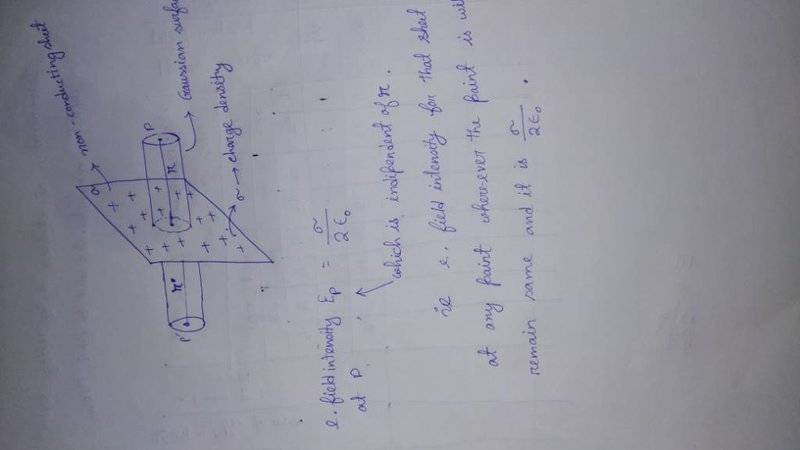# Gauss's law application

• I
• nivamani Rajbongshi
In summary, the electric field intensity at a point on a non-conducting sheet is independent of its distance from the sheet. This can be understood through Gauss' law, which considers the electric flux and the fact that the dimensions of the sheet are much larger than the distance from the sheet. This allows us to treat the sheet as effectively infinite and ignore any effects from being near an edge. Furthermore, since the field at any point is perpendicular to the sheet, the only parts of the Gaussian surface that will contribute to the flux are the end pieces. Therefore, the field does not depend on the distance of the point from the surface of the sheet.f

#### nivamani Rajbongshi

why electric field intensity at a point of a non conducting sheet is independent of r?#### Attachments

why electric field intensity at a point of a non conducting sheet is independent of r?
Do you understand the derivation from Gauss' law? (You can also just add up all the contributions to the electric field at a point. Same result.)

i m confused. can you please explain it?

Last edited by a moderator:
Do you understand the derivation from Gauss' law? (You can also just add up all the contributions to the electric field at a point. Same result.)
Gauss's law is all about the electric flux, isn't it. So the line of force of a charge travel to infinity, so at a point on which we will calculate e.field intensity may lie at infinite distance, also in this derivation we do calculate the derivation by taking 0 rad angle between E and ds, i.e. all the lines of force that we take for calculation are may be perpendicular to that sheet so for those lines of forces the point on which we r going to calculate E doesn't depend on the distance of the point from the surface of the sheet. Am i right?? I don't think...so, Please explain me clearly.

So the line of force of a charge travel to infinity, so at a point on which we will calculate e.field intensity may lie at infinite distance,
Forget about finding the field "at infinity". The point of this model is that the dimensions of the plate are huge compared to the distance from the plate, so that we can treat the plate as effectively infinite (and thus ignore any effects from being near an edge).

also in this derivation we do calculate the derivation by taking 0 rad angle between E and ds, i.e. all the lines of force that we take for calculation are may be perpendicular to that sheet
The field at any point is perpendicular to the sheet. Thus the only parts of the Gaussian surface that will have a flux is the end pieces.

so for those lines of forces the point on which we r going to calculate E doesn't depend on the distance of the point from the surface of the sheet.
Since the amount of charge enclosed -- and thus the flux -- doesn't change with distance from the plate, we can conclude that the field does not depend on that distance.

Thanks i got it...[emoji106][emoji106]

•berkeman